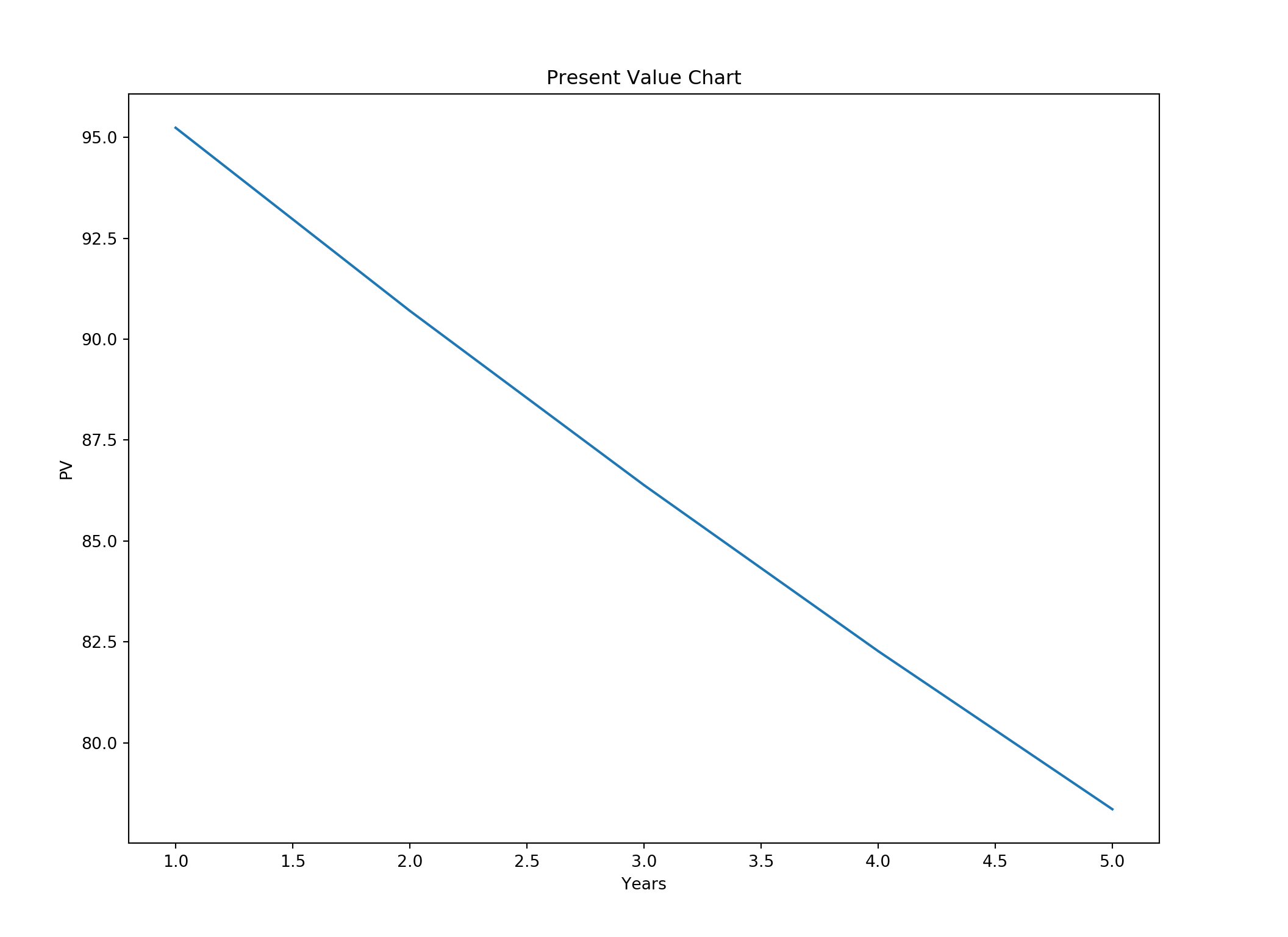In this article we will perform the same time value of money calculations using Python. First let us import the necessary modules/libraries.

``````import pandas as pd
import numpy as np
import matplotlib.pyplot as plt``````

Creating a pandas dataframe.

``````# Setting interest rate to 5%
r = 0.05
cf = pd.DataFrame({'Year':[1,2,3,4,5], "cash_flow":[100,100,100,100,100]})
cf["pv"] = cf['cash_flow'] / (1.0 + r)**cf['Year']
npv = cf["pv"].sum()
print(cf)``````
``````##    Year  cash_flow         pv
## 0     1        100  95.238095
## 1     2        100  90.702948
## 2     3        100  86.383760
## 3     4        100  82.270247
## 4     5        100  78.352617``````
``print(npv)``
``## 432.9476670630819``

### Calculating the Net Present Value (NPV)

``````fig = plt.figure(figsize=(11,8))
ax1.plot(cf["Year"], cf["pv"])
ax1.set_xlabel('Years') # Notice the use of set_ to begin methods
ax1.set_ylabel('PV')
ax1.set_title('Present Value Chart')
plt.show()````````print("The NPV is ", + round(npv))``
``## The NPV is  433.0``

### Calculating net present value of uneven cash flows

``````cf = pd.DataFrame({"Year":[1,2,3,4,5],
"cf":[100,200,300,400,500]})
print(cf)``````
``````##    Year   cf
## 0     1  100
## 1     2  200
## 2     3  300
## 3     4  400
## 4     5  500``````
``````r = 0.05
cf["pv"] = cf["cf"] / (1 + r)**cf["Year"]
npv = cf["pv"].sum()
print(npv)``````
``## 1256.63934364013``

### Calculating the PV with negative cash flow

In many cases we will have an initial cash flow that is negative. Think of this as buying a bond, where we buy a bond for \$250 and it pays an interest of \$100 each year. We can solve the present value of such a bond as below.

``````cf = pd.DataFrame({"Year":[0,1,2,3,4,5],
"cf":[-250,100,100,100,100,250]})
print(cf)``````
``````##    Year   cf
## 0     0 -250
## 1     1  100
## 2     2  100
## 3     3  100
## 4     4  100
## 5     5  250``````
``````r = 0.05
cf["pv"] = cf["cf"] / (1 + r)**cf["Year"]
npv = cf["pv"].sum()
print(npv)``````
``## 300.4765920333507``

### Calculating the present value of a finite annuity

In some cases we have to calculate the present value of stream of equal payments. Many times a lottery winner is given the option to get the money in equal payments or a lump sum. Let us suppose you win a \$50000 lottery and you are given two options

1. Receive five equal payments of \$10000
2. Lump sum \$45000.

Let us suppose the bank pays 5% interest rate on the deposit.

At first glance it may appear that five equal payments of \$10000 is better than \$45000. After all \$50000 is more than \$45000. To solve this problem we can calculate the net present value of those payments as below.

``````pmt = 10000
n = 5
r = 0.05
cf = pd.DataFrame({'period':np.arange(1,6),
"pmt":pmt})
print(cf)``````
``````##    period    pmt
## 0       1  10000
## 1       2  10000
## 2       3  10000
## 3       4  10000
## 4       5  10000``````
``````r = 0.05
cf["pv"] = cf['pmt']/(1 + r) ** cf["period"]
npv = cf["pv"].sum()
print(npv)``````
``## 43294.76670630819``

The net present value of five equal payments is 43294.767. This payment is less that \$45000 offered today. Therefore it is better to take the lump sum payment of \$45000 and deposit it in the bank than five equal payments of \$10000.

### Calculating the present value infinite annuity

Sometimes one can buy an infinite payment stream. Bank of England issued such bonds that paid interest forever. How can we calculate the net present value of such bonds. Let us suppose we are given an offer to buy a bond that pays \$1000 per year in interest forever. If the current interest rate is 5%, how much should we pay for this bond? We can do the calculation as below.

``````cf = 1000
r = 0.05
value = cf/r
print(value)``````
``## 20000.0``

### Calculating present value of finite growing annuity

There are cases when an investor may be offered a stream of payments that grow at a certain rate. Suppose you own an apartment complex. The tenants pay you a fixed rent every month. You can increase the rent to tackle higher inflation. This apartment building may be described as an annuity payment that grows at the rate of inflation. We can calculate the present value of those payments as below.

``````payment = 1000
growth = 0.03 # Growth rate of the payments
n = 5 # number of year
rate = 0.06 # Interest rates
cf = pd.DataFrame({"period":np.arange(1,6),
'cf':payment})
print(cf)``````
``````##    period    cf
## 0       1  1000
## 1       2  1000
## 2       3  1000
## 3       4  1000
## 4       5  1000``````

Next we increase the cash flows at the rate of inflation.

``````cf["cf"] = payment * (1 + growth) ** (cf["period"] - 1)
print(cf)``````
``````##    period          cf
## 0       1  1000.00000
## 1       2  1030.00000
## 2       3  1060.90000
## 3       4  1092.72700
## 4       5  1125.50881``````
``````cf["pv"] = cf["cf"] / (1 + rate) ** cf["period"]
print(cf)``````
``````##    period          cf          pv
## 0       1  1000.00000  943.396226
## 1       2  1030.00000  916.696333
## 2       3  1060.90000  890.752097
## 3       4  1092.72700  865.542132
## 4       5  1125.50881  841.045657``````
``````npv = cf["pv"].sum()
print("The NPV of growing payments is", + npv)``````
``## The NPV of growing payments is 4457.432446253072``

### Calculating present value of infinite growing annuity

In case of infinite payment stream that grows at a certain rate, we simply subtract the growth rate from the current interest rate as shown below.

Suppose you get paid \$100 per year forever, and this payment grows at 3% each year. What is the net present value of this payment stream.

``````payment = 100
growth = 0.03
rate = 0.06
annuity = payment/(rate - growth)
print("The present value of annuity is ", + annuity)``````
``## The present value of annuity is  3333.3333333333335``